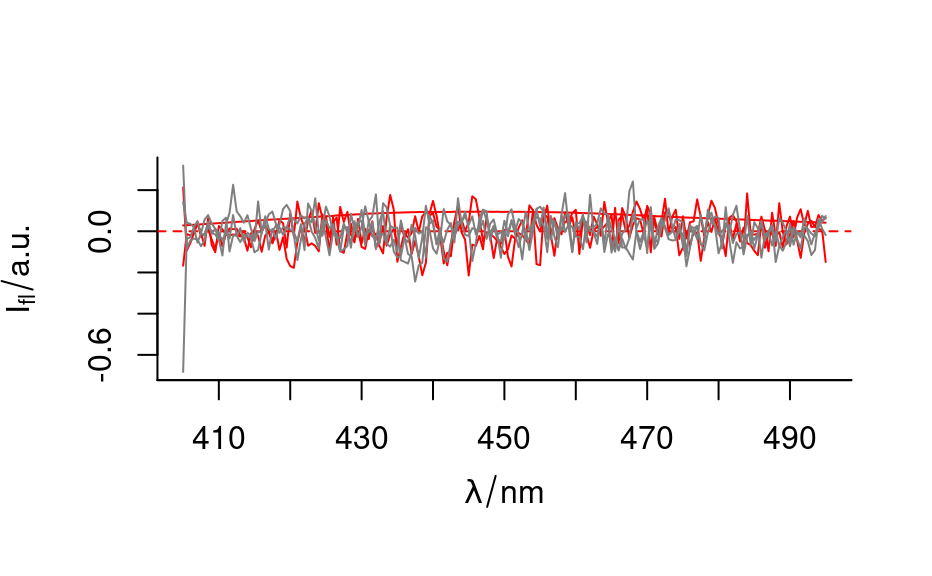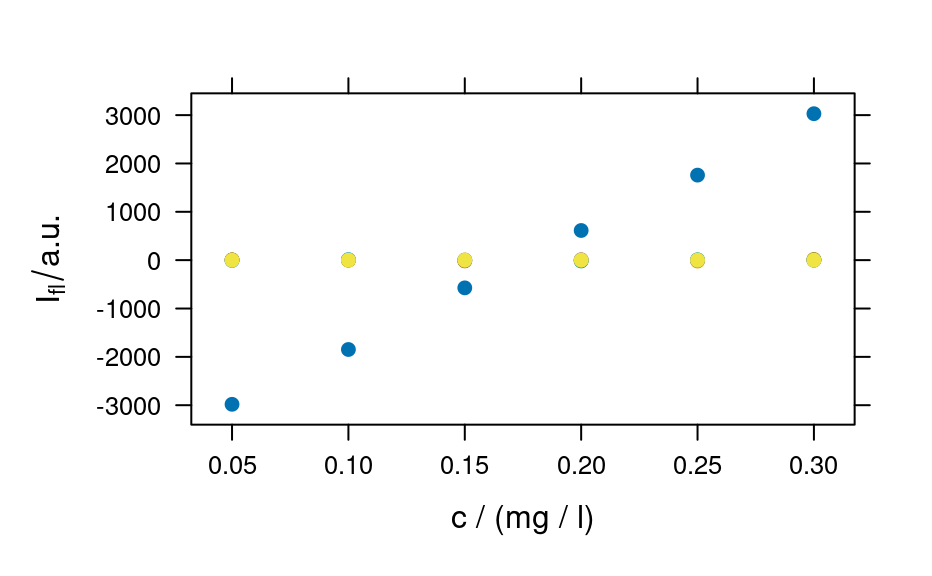Decomposition of the spectra matrix is a common procedure in chemometric data analysis. scores and loadings convert the result matrices into new hyperSpec objects.

decomposition(
object,
x,
wavelength = seq_len(ncol(x)),
label.wavelength,
label.spc,
scores = TRUE,
retain.columns = FALSE,
...
)

Arguments

object

A hyperSpec object.

x

matrix with the new content for object@data$spc. Its size must correspond to rows (for scores) and to either columns or rows (for loadings) of object. wavelength for a scores-like x: the new object@wavelength. label.wavelength The new label for the wavelength axis (if x is scores-like). If not given, the label of object is kept. label.spc The new label for the spectra matrix. If not given, the label of object is kept. scores is x a scores-like matrix? retain.columns for loading-like decompostition (i.e. x holds loadings, pure component spectra or the like), the data columns need special attention. Columns with different values across the rows will be set to NA if retain.columns is TRUE, otherwise they will be deleted. ... ignored. Value A hyperSpec object, updated according to x Details Multivariate data are frequently decomposed by methods like principal component analysis, partial least squares, linear discriminant analysis, and the like. These methods yield latent spectra (or latent variables, loadings, components, ...) that are linear combination coefficients along the wavelength axis and scores for each spectrum and loading. The loadings matrix gives a coordinate transformation, and the scores are values in that new coordinate system. The obtained latent variables are spectra-like objects: a latent variable has a coefficient for each wavelength. If such a matrix (with the same number of columns as object has wavelengths) is given to decomposition (also setting scores = FALSE), the spectra matrix is replaced by x. Moreover, all columns of object@data that did not contain the same value for all spectra are set to NA. Thus, for the resulting hyperSpec object, plot_spc() and related functions are meaningful. plotmap() cannot be applied as the loadings are not laterally resolved. The scores matrix needs to have the same number of rows as object has spectra. If such a matrix is given, decomposition will replace the spectra matrix is replaced by x and object@wavelength by wavelength. The information related to each of the spectra is retained. For such a hyperSpec object, plotmap() and plotc() and the like can be applied. It is also possible to use the spectra plotting, but the interpretation is not that of the spectrum any longer. See also See %*% for matrix multiplication of hyperSpec objects. See e.g. stats::prcomp() and stats::princomp() for principal component analysis, and package pls for Partial Least Squares Regression. Author C. Beleites Examples pca <- prcomp(flu) pca.loadings <- decomposition(flu, t(pca$rotation), scores = FALSE)
pca.center <- decomposition(flu, pca$center, scores = FALSE) pca.scores <- decomposition(flu, pca$x)

plot(pca.center)